Courses

# NCERT Exemplar - Sound Class 9 Notes | EduRev

## Class 9 : NCERT Exemplar - Sound Class 9 Notes | EduRev

The document NCERT Exemplar - Sound Class 9 Notes | EduRev is a part of the Class 9 Course Science Class 9.
All you need of Class 9 at this link: Class 9

Q.1. The given graph shows the displacement versus time relation for a disturbance travelling with a velocity of 1500 ms-1. Calculate the wavelength of the disturbance.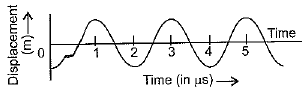Ans: From the graph, T = 2 x 10-6 s,
speed (v) = 1500 ms-1
∴ Wave Length (λ) = v x T
= 1500 x 2 x 10-6
= 3 x 10-3 m

Q.2. Which of the below two graphs (a) and (b) representing the human voice is likely to be the mate voice? Give reason for your answer.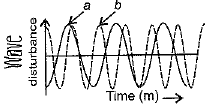Ans: Graph (a) represents the male voice. Usually the male voice has less pitch (or frequency) as compared to female.

Q.3. Why do we hear the sound produced by the humming bees while the sound of vibrations of pendulum is not heard?
Ans: The frequency of oscillations of a pendulum is below the minimum audible value i.e., 20 Hz, while the frequency of the sound of the humming bees is in the audible range.

Q.4. If any explosion takes place at the bottom of a lake, what type of shock waves in water will be produced?
Ans: Longitudinal waves will be produced.

Q.5. The sound produced by a thunderstorm is heard 10s after the lightning is seen. Calculate the approximate distance of the thunder cloud. (Given speed of sound = 340 ms-1.)
Ans:  v = 340 ms-1, t = 10 s.
Distance (d) = speed (v) x time (t) = 340 x 10 = 3.4 km

Q.6. For hearing the loudest ticking sound heard by the ear, find the angle x in the figure.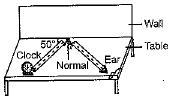Ans:
The angle x = 40° as the angle of reflection and incidence are to be equal.

Q.7. Represent graphically by two separate diagrams in each case.
(a) Two sound waves having the same amplitude but different frequencies.
(b) Two sound waves having the same frequency but different amplitudes.
(c) Two sound waves having different amplitudes and also different wavelengths.
Ans: (a)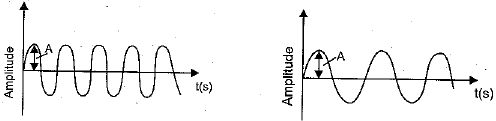(b)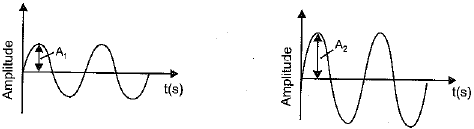(c)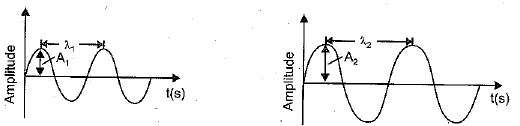Q.8. Establish the relationship between speed of sound, its wavelength and frequency. If velocity of sound in air is 340 ms-1, calculate
(a) wavelength when frequency is 256 Hz.
(b) frequency when wavelength is 0.85 m.
Ans:  We know that speed of the wave,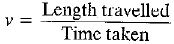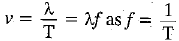v = 340 ms-1
(a) λ =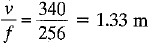(b) f =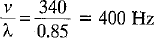Q.9. Draw a curve showing density or pressure variations with respect to distance for a disturbance produced by sound. Mark the position of compression and rarefaction on this curve. Also, define wavelength and time period using this curve.
Ans: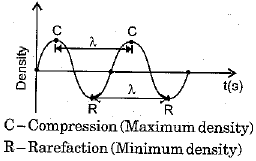Wavelength (λ) is defined as the separation between two points in the same phase in two consecutive waves, i.e. (C - C) or (R - R).
The time taken for one complete oscillation is called time period, i.e. time for C - C or R - R.

Offer running on EduRev: Apply code STAYHOME200 to get INR 200 off on our premium plan EduRev Infinity!

## Science Class 9

95 videos|223 docs|135 tests

,

,

,

,

,

,

,

,

,

,

,

,

,

,

,

,

,

,

,

,

,

;